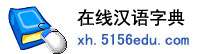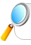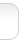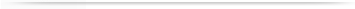汉字   按部首检索  按拼音检索陈铿 林铿 杨铿 郑铿 李铿 吴铿 黄铿 邓铿 高铿 姚铿 汪铿 冯铿 朱铿 卢铿 邹铿 田铿 梁铿 涂铿 周铿 王铿 赵铿 郭铿 奉铿

 铿字的男姓名 | 铿字的女姓名 | 铿开头的姓名 | 铿结尾的姓名 | 更多带铿的姓名
 铿 (男) 铿 (女) 文铿 (男) 国铿 (男) 湛铿 (男) 志铿 (男) 尤铿 (男) 祖铿 (男) 秉铿 (男) 锡铿 (男) 荣铿 (男) 子铿 (男) 明铿 (男) 铿梁 (男) 书铿 (男) 延铿 (男) 孝铿 (男) 善铿 (男) 铿锋 (男) 之铿 (男) 智铿 (男) 传铿 (男) 士铿 (男) 世铿 (男) 铿浩 (男) 保铿 (男) 满铿 (男) 铿铿 (女) 鸿铿 (男) 小铿 (男) 立铿 (男) 文铿 (女) 俊铿 (男) 陈铿 (男) 顺铿 (男) 良铿 (女) 南铿 (男) 铿华 (男) 铿栋 (男) 祥铿 (男) 光铿 (男) 庭铿 (男) 铿锴 (男) 铁铿 (男) 铭铿 (男) 绍铿 (男) 江铿 (男) 泽铿 (男) 渊铿 (男) 铿明 (男) 铿攒 (男) 维铿 (男) 铿炉 (男) 继铿 (男) 铿骏 (男) 以铿 (男) 铿燃 (男) 祺铿 (男) 承铿 (男) 铿文 (男) 土铿 (男) 铿新 (男) 铭铿 (女) 铿周 (男) 峥铿 (男) 家铿 (男) 伟铿 (男) 国铿 (女) 润铿 (男) 炊铿 (男) 邦铿 (男) 铿假 (男) 日铿 (男) 迪铿 (男) 钰铿 (男) 从铿 (男) 铿翩 (男) 友铿 (男) 作铿 (男) 广铿 (男) 铁铿 (女) 敏铿 (男) 东铿 (男) 奕铿 (男) 卫铿 (男) 甲铿 (男) 铿烁 (男) 琳铿 (女) 铿定 (男) 海铿 (男) 铿阳 (女) 宜铿 (男) 政铿 (男) 键铿 (男)

 版权所有　在线汉语字典   浙ICP备05019169号